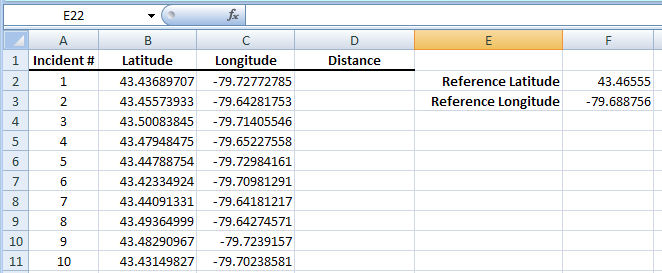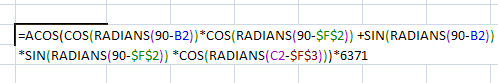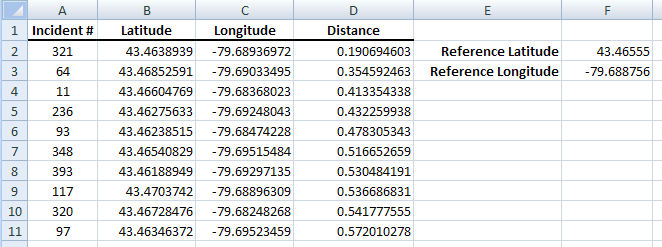### The Poor Man’s Proximity Tool: The Haversine Formula

The haversine formula is a method for finding the great-circle distance between a pair of latitude-longitude coordinates. In crime analysis I use the formula for finding incidents that have taken place within a particular distance, for example within 500 meters, of a location.

My approach uses Excel to calculate the haversine distance between a reference location and the latitude-longitude of every other location in my spreadsheet. I then sort the results to find the locations that are within the distance that I’m interested in. I’ve worked the following example in Excel to illustrate the method.

Imagine that you have a list of 1000 occurrences with the latitudes and longitudes of each of the occurrence locations. Now also imagine that you have a reference location and that you want to determine how many of the 1000 incidents occurred within 500 meters of the reference location. I set my spreadsheet up like this:Note that in column B I have entered all of the latitudes and in column C I have entered all the longitudes. I have also entered my reference latitude and longitude in cells E2 and E3 so that they can be referenced by the haversine formula. Also note that both latitudes and longitudes are decimal degrees as opposed to degree minutes seconds.

In column D I enter the Excel-ified version of the haversine formula which I have reproduced below. It gets a bit complex because Excel likes to deal with radians as opposed to degrees when using the COS and SIN functions so it is necessary to use Excel’s RADIANS function to convert the latitudes and longitudes. The final multiplication by 6371 represents the Earth’s radius in kilometres.A note for pedants: I realize that the Earth is not a perfect sphere and that means that the calculated distances will be somewhat off if you are dealing with large distances. But, for the distances we are concerned with–distances of 10s of kilometres–the impact is negligible and can be ignored.

By filling column D with the expression I calculate the separation distance between the reference point and each incident. Now, if the distance column is sorted from smallest to largest I can easily see the incidents that occurred within 500 meters (or 0.5 kilometres) of the reference location.I find this method useful for determining the proximity of all sorts of things. For example, if the reference coordinates are for an intersection the method can be used to find all motor vehicle collisions that occurred within 100 meters. Or say there was a break and enter, a proximity search can be done against field contacts that occurred within 1 kilometre of the occurrence address. Really anything that has a recorded latitude and longitude can be used. Best of all, it doesn’t require a GIS, just Excel and the formula.